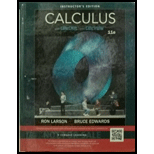Chapter 8.8, Problem 87E### Calculus (MindTap Course List)

11th Edition
Ron Larson + 1 other
ISBN: 9781337275347

#### Solutions

Chapter
Section### Calculus (MindTap Course List)

11th Edition
Ron Larson + 1 other
ISBN: 9781337275347
Textbook Problem

# Comparing Integrals(a) Show that ∫ − ∞ ∞ sin x   d x diverges.(b) Show that lim a → ∞ ∫ − a a sin x   d x = 0 .(c) What do parts (a) and (b) show about the definition of improper integrals?

(a)

To determine

To prove: The integral sinxdx diverges.

Explanation

Given: sinxdx

Formula used:

sinxdx=cosx+c

The limit formula for continuous functionis

limxcf(x)=f(c)

Proof:

The given integral can be solved as given below.

sinxdx=0sinxdx+0sinxdx=limaa0sinxdx+lim

(b)

To determine

To prove: The limit limaaasinxdx=0.

(c)

To determine
The information about improper integrals as given by parts (a) and (b).

### Still sussing out bartleby?

Check out a sample textbook solution.

See a sample solution

#### The Solution to Your Study Problems

Bartleby provides explanations to thousands of textbook problems written by our experts, many with advanced degrees!

Get Started

## Additional Math Solutions

#### Determine the infinite limit. limx2xcscx

Single Variable Calculus: Early Transcendentals, Volume I

#### let f(x) = x 1, g(x) = x+1, and h(x) = 2x3 1. Find the rule for each function. 11. fg

Applied Calculus for the Managerial, Life, and Social Sciences: A Brief Approach

#### In problems 63-73, factor each expression completely. 71.

Mathematical Applications for the Management, Life, and Social Sciences

#### Solve for y:5x8y=10

Elementary Technical Mathematics